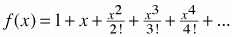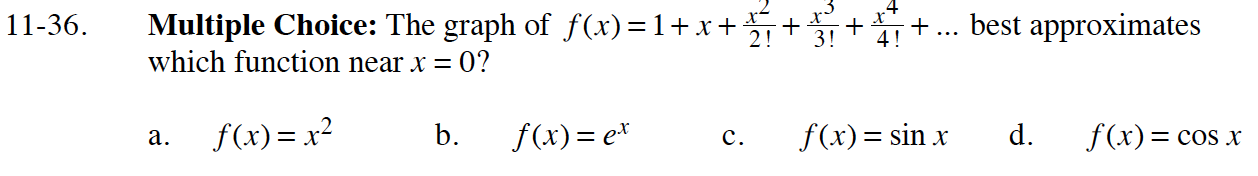### Home > CALC > Chapter 11 > Lesson 11.1.3 > Problem11-36

11-36.
1. Multiple Choice: The graph ofbest approximates which function near x = 0? Homework Help ✎

1. f(x) = x2

2. f(x) = ex

3. f(x) = sin x

4. f(x) = cos xf(0) = 1
Which choices match this situation?

f′(0) = 1
Which choice matches this situation?